# 专门为初学者编写的正则表达式入门教程

更新时间：2020年05月09日 00:02:05   作者：手边字节

### 1 介绍

• 输入验证
• 查找替换操作
• 高级字符串操作
• 文件搜索或重命名
• 白名单和黑名单

• XML 或 HTML 解析
• 完全匹配的日期

### 2 基础

• `/p/g`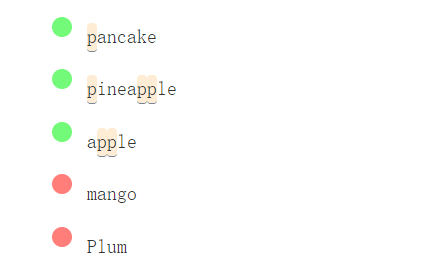• `/pp/g`### 3 字符组

• `/[aeiou]/g``[aeiou]/g` 匹配输入字符串中的所有元音。

• `/p[aeiou]t/g`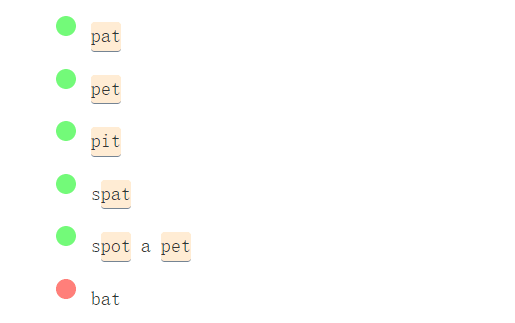• `/[a-z]/g`• `/[A-Za-z0-9_-]/g`• `A-Z`
• `a-z`
• `0-9`
• `_` 或者 `-`

• `/[^aeiou]/g``/[aeiou]/g``/[^aeiou]/g` 之间的唯一区别是 ^ 紧跟在左括号之后。其目的是"否定"括号中定义的规则。它表示的意思是：

#### 3.1 例子

• `/[^a-zA-Z_0-9-]/g`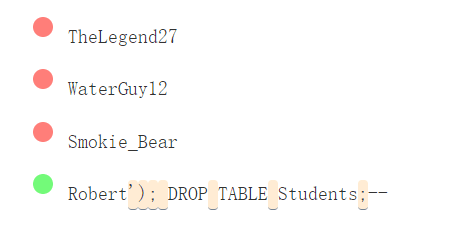`/[A-HJ-NP-Za-kmnp-z2-9]/g`### 4 字符转义

#### 4.1 数字字符 `\d`

• `/\d/g` (这里请仔细看)• `/\d\d/g``\D``\d` 的反面，相当于`[^0-9]`

• `/\D/g`#### 4.2 单词字符 `\w`

• 小写字母 a-z
• 大写字母 A-Z
• 数字 0-9
• 下划线 _

• `/\w/g`• `/\W/g`#### 4.3 空白字符 `\s`

• 空格
• tab 制表符 `\t`
• 回车 `\r`
• 换行符 `\n`
• 换页 `\f`

• `/\s/g`• `/\S/g` (大写 s)#### 4.4 任意字符 `.`

• `/./g`### 5 转义

• `|`
• `{,}`
• `(,）`
• `[,]`
• `^`, `\$`
• `+`, `*`, `?`
• `\`
• `.` 只在字符类中的字面量。
• `-` : 有时是字符类中的特殊字符。

• `/\(paren\)/g`• `/(paren)/g`• `/example\.com/g`• `/example.com/g`• `/A\+/g`• `/A+/g`• `/worth \\$5/g`• `/worth \$5/g`#### 5.1 例子

JavaScript 内联注释

• `/\/\/.*`• `/*[^\*]*\*`### 6 组

• 提取匹配的子集
• 重复分组任意次数
• 参考先前匹配的子字符串
• 增强可读性
• 允许复杂的替换

#### 6.1 捕获组

• `/a(bcd)e/g`• `/\{([^{}]*)\}/g`• `/a(bcd)+e/g`• `/(\d\d\d\d)-W(\d\d)/g`#### 6.2 回溯

• `/([abc])×\1×\1/g`• `/[abc][abc][abc]/g`• `/[abc]\1\1/g`• `/\w+([,|])\w+\1\w+/g`• `/\w+[,|]\w+[,|]\w+/g`#### 6.4 例子

• `/^\?(\w+)=(\w+)(?:&(\w+)=(\w+))*\$/g`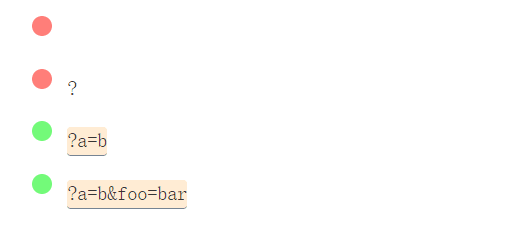(基础的) HTML 标签

• `/<([a-z]+)+>(.*)<\/\1>/gi`John Doe
Jane Doe
Sven Svensson
Janez Novak
Janez Kranjski
Tim Joe

Doe, John
Doe, Jane
Svensson, Sven
Novak, Janez
Kranjski, Janez
Joe, Tim

```This is a paragraph with some words. Some instances of the word "word" are in their plural form: "words". ```

`This is a paragraph with some phrases. `

`Yet, some are in their singular form: "phrase". `

### 7 重复

#### 7.1 可选项

• `/a?/g`• `/https?/g`• `/url: (www\.)?example\.com/g`#### 7.2 零次或者多次

• `/a*/g`#### 7.3 一次或者多次

• `/a+/g`#### 7.4 精确的 x 次

• `/a{3}/g`• `/#[0-9A-F]{6}/g`#### 7.5 最小次和最大次之间

• `/a{2,4}/g`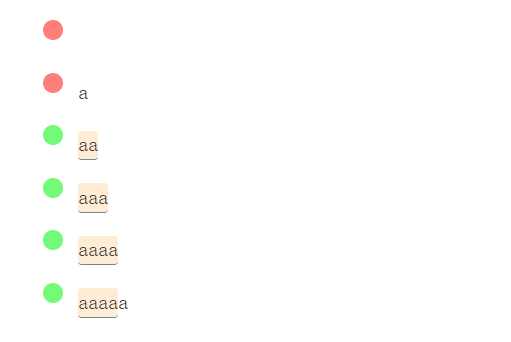`{min,max}` 中逗号后面不要有空格。

#### 7.6 最少 x 次

• `/a{2,}/g`#### 7.7 贪婪模式的注意事项

• `/a*/g`• `/".*"/g`• `/".*?"/g`• `/"[^"]*"/g`-Andrew S on StackOverflow

• `/<.+>/g`• `/<.+?>/g`#### 7.8 例子

• `/([a-km-zA-HJ-NP-Z0-9]{26,33})/g` (思考： {26,33}？呢)• `/(?:https?:\/\/)?(?:www\.)?youtube\.com\/watch\?.*?v=([^&\s]+).*/gm`### 8 交替

• `/foo|bar|baz/g`• `/Try (foo|bar|baz)/g`Try 后面跟着 foo, bar, 和 baz 中的一个。

• `/1\d\d|2[0-4]\d|250/g`#### 例子

• `/#[0-9A-F]{6}|[0-9A-F]{3}``[0-9A-F]{6}` 要放在`[0-9A-F]{3}`的前面，这一点非常重要。否则：

• `/#([0-9A-F]{3}|[0-9A-F]{6})/g`• `/^M{0,4}(CM|CD|D?C{0,3})(XC|XL|L?X{0,3})(IX|IV|V?I{0,3})\$/g`### 9 修饰符

#### 9.1 全局修饰符（g)

• `/[aeiou]/g`• `/[aeiou]/`#### 9.2 不区分大小写修饰符（i)

• `/#[0-9A-F]{6}/i`• `/#[0-9A-F]{6}/`• `/#[0-9A-Fa-f]{6}/`#### 9.3 多行模式修饰符（m)

`bar foo baz `

#### 9.4 Dot-all修饰符 (s)

ES2018 之前的 JavaScript 不支持这个修饰符。 Ruby 也不支持这个修饰，而是用 m 表示。

`.`通常匹配除换行符以外的任何字符。使用dot all修饰符后，它也可以匹配换行符。

### 10 锚点

#### 10.1 行首 `^`

• `/^p/g`#### 10.2 行尾

• `/p\$/g`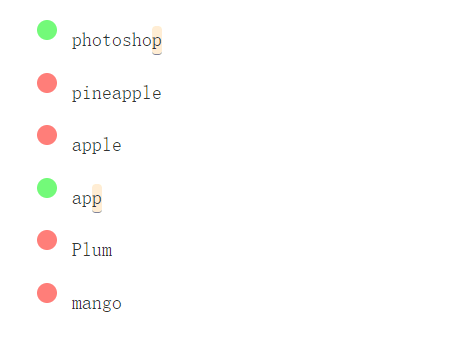`^``\$`锚点经常一起使用，以确保正则和字符串整个匹配，而不仅仅是部分匹配。

• `/^p\$/g`• `/^https?\$/g`#### 10.3 字边界 `\b`

• `/\bp/g`• `/\bp\b/g`• `/\bcat\b/g`• `/\Bp/g`• `/\Bp\B/g`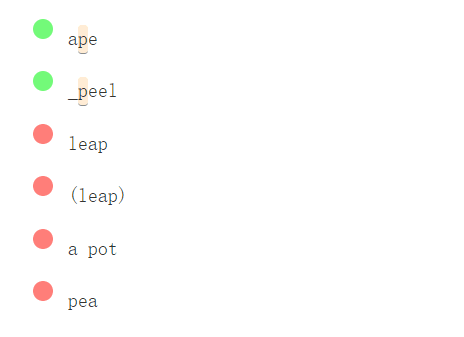`^…\$``\b…\b`是常见的模式，您几乎总是需要这 2 个防止意外匹配。

#### 10.4 例子

• `/\s+\$/gm`markdown 标题

• `/^## /gm`• `/## /gm`### 11 零宽断言（lookaround)

• 正向`(?=…)`
• 负向`（?!…)`
• 先行断言（lookbehind)
• 正向`(?<=…)`
• 负向`（?<!…)`

• `/_(?=[aeiou])/g`• `/(.+)_(?=[aeiou])(?=\1)/g`• `/(?=.*#).*/g`• `/_(?![aeiou])/g`• `/^(?!.*#).*\$/g`• `/foo(?!bar)/g`• `/---(?:(?!---).)*---/g`#### 11.2 例子

`/^(?=.*\d)(?=.*[a-z])(?=.*[A-Z])(?=.*[a-zA-Z]).{8,}\$/`• `/(['"])(?:(?!\1).)*\1/g`• `/(['"])[^'"]*\1/g`### 12 进阶例子

JavaScript 注释

• `/\/\*[\s\S]*?\*\/|\/\/.*/g``[\s\S]`是一种匹配任何字符（包括换行符）的技巧。我们避免使用dot-all 修饰符，因为我们需要使用`.` 表示单行注释。

24小时时间

• `/^(?[0-9]|2[0-3]):[0-5][0-9](:[0-5][0-9])?\$/g`IP 地址

• `/\b(?:(?:2(?:[0-4][0-9]|5[0-5])|[0-1]?[0-9]?[0-9])\.){3}(?:(?:2([0-4][0-9]|5[0-5])|[0-1]?[0-9]?[0-9]))\b/g`• `/<Example source="(.*?)" flags="(.*?)">/gm`• 可选符号

• 可选整数部分

• 可选小数部分

• 可选指数部分

• `/^([+-]?(?=\.\d|\d)(?:\d+)?(?:\.?\d*))(?:[eE]([+-]?\d+))?\$/g`HSL颜色

• `/^0*(?:360|3[0-5]\d|?\d?\d)\$/g`• `/^(?:100(?:\.0+)?|\d?\d(?:\.\d+)?)%\$/g`HSL 和 百分比

• `/^hsl\(\s*0*(?:360|3[0-5]\d|?\d?\d)\s*(?:,\s*0*(?:100(?:\.0+)?|\d?\d(?:\.\d+)?)%\s*){2}\)\$/gi`